# Test: Syllogism- 3

## 30 Questions MCQ Test General Intelligence and Reasoning for SSC CHSL | Test: Syllogism- 3

Description
Attempt Test: Syllogism- 3 | 30 questions in 30 minutes | Mock test for CAT preparation | Free important questions MCQ to study General Intelligence and Reasoning for SSC CHSL for CAT Exam | Download free PDF with solutions
QUESTION: 1

### Directions to Solve In each of the following questions two statements are given and these statements are followed by two conclusions numbered (1) and (2). You have to take the given two statements to be true even if they seem to be at variance from commonly known facts. Read the conclusions and then decide which of the given conclusions logically follows from the two given statements, disregarding commonly known facts. Give answer: (A) If only (1) conclusion follows (B) If sonly (2) conclusion follows (C) If either (1) or (2) follows (D) If neither (1) nor (2) follows and (E) If both (1) and (2) follow. Question - Statements: Some actors are singers. All the singers are dancers. Conclusions: Some actors are dancers. No singer is actor.

Solution: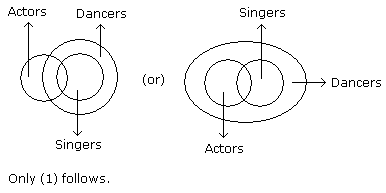QUESTION: 2

### Directions to Solve In each of the following questions two statements are given and these statements are followed by two conclusions numbered (1) and (2). You have to take the given two statements to be true even if they seem to be at variance from commonly known facts. Read the conclusions and then decide which of the given conclusions logically follows from the two given statements, disregarding commonly known facts. Give answer: (A) If only (1) conclusion follows (B) If sonly (2) conclusion follows (C) If either (1) or (2) follows (D) If neither (1) nor (2) follows and (E) If both (1) and (2) follow. Question - Statements: All the harmoniums are instruments. All the instruments are flutes. Conclusions: All the flutes are instruments. All the harmoniums are flutes.

Solution: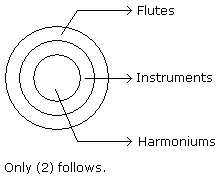QUESTION: 3

### Directions to Solve In each of the following questions two statements are given and these statements are followed by two conclusions numbered (1) and (2). You have to take the given two statements to be true even if they seem to be at variance from commonly known facts. Read the conclusions and then decide which of the given conclusions logically follows from the two given statements, disregarding commonly known facts. Give answer: (A) If only (1) conclusion follows (B) If sonly (2) conclusion follows (C) If either (1) or (2) follows (D) If neither (1) nor (2) follows and (E) If both (1) and (2) follow. Question - Statements: Some mangoes are yellow. Some tixo are mangoes. Conclusions: Some mangoes are green. Tixo is a yellow.

Solution: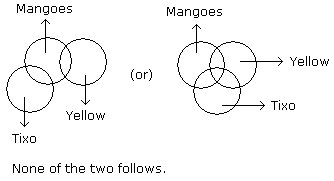QUESTION: 4

Directions to Solve

In each of the following questions two statements are given and these statements are followed by two conclusions numbered (1) and (2). You have to take the given two statements to be true even if they seem to be at variance from commonly known facts. Read the conclusions and then decide which of the given conclusions logically follows from the two given statements, disregarding commonly known facts.

• (A) If only (1) conclusion follows
• (B) If sonly (2) conclusion follows
• (C) If either (1) or (2) follows
• (D) If neither (1) nor (2) follows and
• (E) If both (1) and (2) follow.

Question -

Statements: Some papers are pens. All the pencils are pens.

Conclusions:

1. Some pens are pencils.
2. Some pens are papers.
Solution: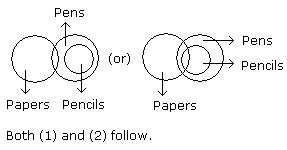QUESTION: 5

Directions to Solve

In each of the following questions two statements are given and these statements are followed by two conclusions numbered (1) and (2). You have to take the given two statements to be true even if they seem to be at variance from commonly known facts. Read the conclusions and then decide which of the given conclusions logically follows from the two given statements, disregarding commonly known facts.

• (A) If only (1) conclusion follows
• (B) If sonly (2) conclusion follows
• (C) If either (1) or (2) follows
• (D) If neither (1) nor (2) follows and
• (E) If both (1) and (2) follow.

Question -

Statements: Some ants are parrots. All the parrots are apples.

Conclusions:

1. All the apples are parrots.
2. Some ants are apples.
Solution: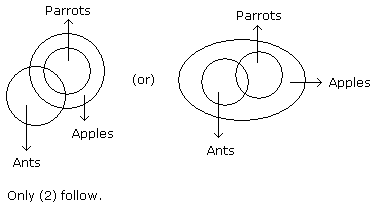QUESTION: 6

Directions to Solve

In each of the following questions two statements are given and these statements are followed by two conclusions numbered (1) and (2). You have to take the given two statements to be true even if they seem to be at variance from commonly known facts. Read the conclusions and then decide which of the given conclusions logically follows from the two given statements, disregarding commonly known facts.

• (A) If only (1) conclusion follows
• (B) If sonly (2) conclusion follows
• (C) If either (1) or (2) follows
• (D) If neither (1) nor (2) follows and
• (E) If both (1) and (2) follow.

Question -

Statements: All the actors are girls. All the girls are beautiful.

Conclusions:

1. All the actors are beautiful.
2. Some girls are actors.
Solution: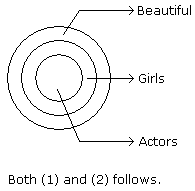QUESTION: 7

Directions to Solve

In each of the following questions two statements are given and these statements are followed by two conclusions numbered (1) and (2). You have to take the given two statements to be true even if they seem to be at variance from commonly known facts. Read the conclusions and then decide which of the given conclusions logically follows from the two given statements, disregarding commonly known facts.

• (A) If only (1) conclusion follows
• (B) If sonly (2) conclusion follows
• (C) If either (1) or (2) follows
• (D) If neither (1) nor (2) follows and
• (E) If both (1) and (2) follow.

Question -

Statements: All the windows are doors. No door is a wall.

Conclusions:

1. Some windows are walls.
2. No wall is a door.
Solution: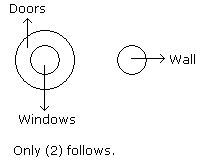QUESTION: 8

Directions to Solve

In each of the following questions two statements are given and these statements are followed by two conclusions numbered (1) and (2). You have to take the given two statements to be true even if they seem to be at variance from commonly known facts. Read the conclusions and then decide which of the given conclusions logically follows from the two given statements, disregarding commonly known facts.

• (A) If only (1) conclusion follows
• (B) If sonly (2) conclusion follows
• (C) If either (1) or (2) follows
• (D) If neither (1) nor (2) follows and
• (E) If both (1) and (2) follow.

Question -

Statements: All cups are books. All books are shirts.

Conclusions:

1. Some cups are not shirts.
2. Some shirts are cups.
Solution: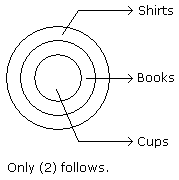QUESTION: 9

Directions to Solve

In each of the following questions two statements are given and these statements are followed by two conclusions numbered (1) and (2). You have to take the given two statements to be true even if they seem to be at variance from commonly known facts. Read the conclusions and then decide which of the given conclusions logically follows from the two given statements, disregarding commonly known facts.

• (A) If only (1) conclusion follows
• (B) If sonly (2) conclusion follows
• (C) If either (1) or (2) follows
• (D) If neither (1) nor (2) follows and
• (E) If both (1) and (2) follow.

Question -

Statements: Some cows are crows. Some crows are elephants.

Conclusions:

1. Some cows are elephants.
2. All crows are elephants.
Solution: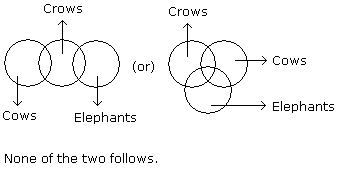QUESTION: 10

Directions to Solve

In each of the following questions two statements are given and these statements are followed by two conclusions numbered (1) and (2). You have to take the given two statements to be true even if they seem to be at variance from commonly known facts. Read the conclusions and then decide which of the given conclusions logically follows from the two given statements, disregarding commonly known facts.

• (A) If only (1) conclusion follows
• (B) If sonly (2) conclusion follows
• (C) If either (1) or (2) follows
• (D) If neither (1) nor (2) follows and
• (E) If both (1) and (2) follow.

Question -

Statements: All the pencils are pens. All the pens are inks.

Conclusions:

1. All the pencils are inks.
2. Some inks are pencils.
Solution: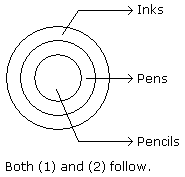QUESTION: 11

Directions to Solve

In each of the following questions two statements are given and these statements are followed by two conclusions numbered (1) and (2). You have to take the given two statements to be true even if they seem to be at variance from commonly known facts. Read the conclusions and then decide which of the given conclusions logically follows from the two given statements, disregarding commonly known facts.

• (A) If only (1) conclusion follows
• (B) If sonly (2) conclusion follows
• (C) If either (1) or (2) follows
• (D) If neither (1) nor (2) follows and
• (E) If both (1) and (2) follow.

Question -

Statements: Some dogs are bats. Some bats are cats.

Conclusions:

1. Some dogs are cats.
2. Some cats are dogs.
Solution: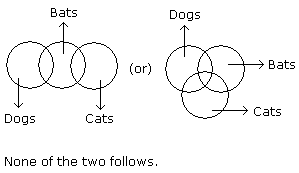QUESTION: 12

Directions to Solve

In each of the following questions two statements are given and these statements are followed by two conclusions numbered (1) and (2). You have to take the given two statements to be true even if they seem to be at variance from commonly known facts. Read the conclusions and then decide which of the given conclusions logically follows from the two given statements, disregarding commonly known facts.

• (A) If only (1) conclusion follows
• (B) If sonly (2) conclusion follows
• (C) If either (1) or (2) follows
• (D) If neither (1) nor (2) follows and
• (E) If both (1) and (2) follow.

Question -

Statements: All the trucks are flies. Some scooters are flies.

Conclusions:

1. All the trucks are scooters.
2. Some scooters are trucks.
Solution: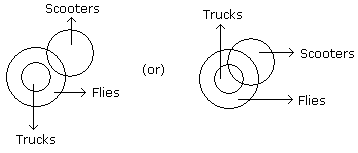Neither (1) nor (2) follows.

QUESTION: 13

Directions to Solve

In each of the following questions two statements are given and these statements are followed by two conclusions numbered (1) and (2). You have to take the given two statements to be true even if they seem to be at variance from commonly known facts. Read the conclusions and then decide which of the given conclusions logically follows from the two given statements, disregarding commonly known facts.

• (A) If only (1) conclusion follows
• (B) If sonly (2) conclusion follows
• (C) If either (1) or (2) follows
• (D) If neither (1) nor (2) follows and
• (E) If both (1) and (2) follow.

Question -

Statements: All buildings are chalks. No chalk is toffee.

Conclusions:

1. No building is toffee
2. All chalks are buildings.
Solution: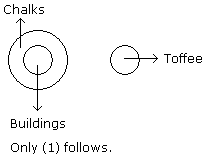QUESTION: 14

Directions to Solve

In each of the following questions two statements are given and these statements are followed by two conclusions numbered (1) and (2). You have to take the given two statements to be true even if they seem to be at variance from commonly known facts. Read the conclusions and then decide which of the given conclusions logically follows from the two given statements, disregarding commonly known facts.

• (A) If only (1) conclusion follows
• (B) If sonly (2) conclusion follows
• (C) If either (1) or (2) follows
• (D) If neither (1) nor (2) follows and
• (E) If both (1) and (2) follow.

Question -

Statements: All cars are cats. All fans are cats.

Conclusions:

1. All cars are fans.
2. Some fans are cars.
Solution: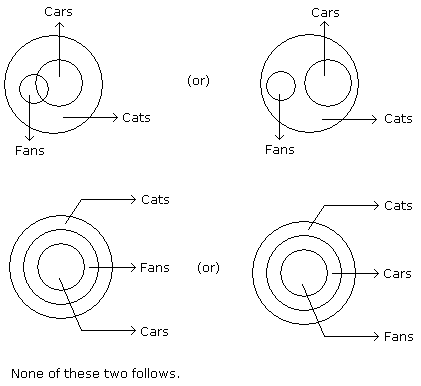QUESTION: 15

Directions to Solve

In each of the following questions two statements are given. Which are followed by four conclusions (1), (2), (3) and (4). Choose the conclusions which logically follow from the given statements.

Question -

Statements: No door is dog. All the dogs are cats.

Conclusions:

1. No door is cat.
2. No cat is door.
3. Some cats are dogs.
4. All the cats are dogs.
Solution: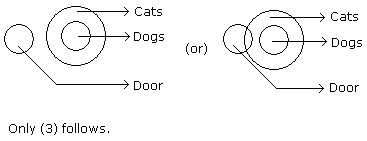QUESTION: 16

Directions to Solve

In each of the following questions two statements are given. Which are followed by four conclusions (1), (2), (3) and (4). Choose the conclusions which logically follow from the given statements.

Question -

Statements: All green are blue. All blue are white.

Conclusions:

1. Some blue are green.
2. Some white are green.
3. Some green are not white.
4. All white are blue.
Solution: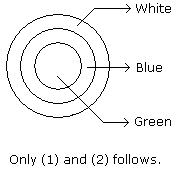QUESTION: 17

Directions to Solve

In each of the following questions two statements are given. Which are followed by four conclusions (1), (2), (3) and (4). Choose the conclusions which logically follow from the given statements.

Question -

Statements: All men are vertebrates. Some mammals are vertebrates.

Conclusions:

1. All men are mammals.
2. All mammals are men.
3. Some vertebrates are mammals.
4. All vertebrates are men.
Solution: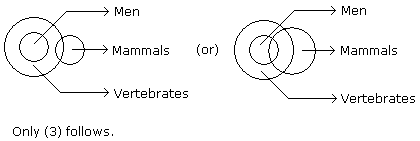QUESTION: 18

Directions to Solve

In each of the following questions two statements are given. Which are followed by four conclusions (1), (2), (3) and (4). Choose the conclusions which logically follow from the given statements.

Question -

Statements: All the phones are scales. All the scales are calculators.

Conclusions:

1. All the calculators are scales.
2. All the phones are calculators
3. All the scales are phones.
4. Some calculators are phones.
Solution: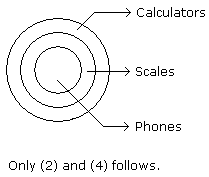QUESTION: 19

Directions to Solve

In each of the following questions two statements are given. Which are followed by four conclusions (1), (2), (3) and (4). Choose the conclusions which logically follow from the given statements.

Question -

Statements: Some tables are T.V. Some T.V. are radios.

Conclusions:

3. All the radios are T.V.
4. All the T.V. are tables.
Solution: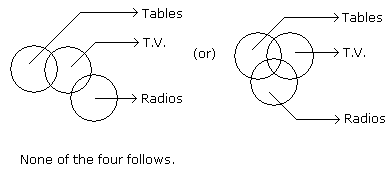QUESTION: 20

Directions to Solve

In each of the following questions two statements are given. Which are followed by four conclusions (1), (2), (3) and (4). Choose the conclusions which logically follow from the given statements.

Question -

Statements: Some pens are books. Some books are pencils.

Conclusions:

1. Some pens are pencils.
2. Some pencils are pens.
3. All pencils are pens.
4. All books are pens.
Solution: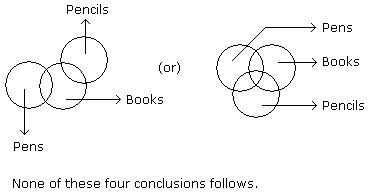QUESTION: 21

Directions to Solve

In each of the following questions two statements are given. Which are followed by four conclusions (1), (2), (3) and (4). Choose the conclusions which logically follow from the given statements.

Question -

Statements: All the goats are tigers. All the tigers are lions.

Conclusions:

1. All the goats are lions.
2. All the lions are goats.
3. Some lions are goats.
4. Some tigers are goats.
Solution: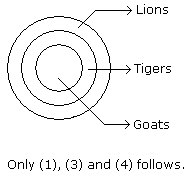QUESTION: 22

Directions to Solve

In each of the following questions two statements are given. Which are followed by four conclusions (1), (2), (3) and (4). Choose the conclusions which logically follow from the given statements.

Question -

Statements: All the books are pencils. No pencil is eraser.

Conclusions:

1. All the pencils are books.
2. Some erasers are books.
3. No book is eraser.
4. Some books are erasers.
Solution: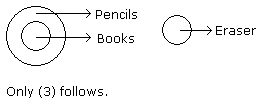QUESTION: 23

Directions to Solve

In each of the following questions two statements are given. Which are followed by four conclusions (1), (2), (3) and (4). Choose the conclusions which logically follow from the given statements.

Question -

Statements: All the research scholars are psychologists. Some psychologists are scientists.

Conclusions:

1. All the research scholars are scientists.
2. Some research scholars are scientists.
3. Some scientists are psychologists.
4. Some psychologists are research scholars.
Solution: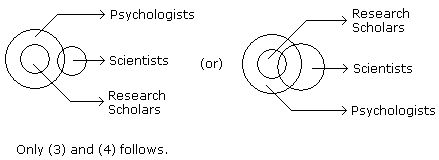QUESTION: 24

Directions to Solve

In each of the following questions there are three statements. Which are followed by three or four conclusions. Choose the conclusions which logically follow from the given statements.

Question -

Statements: All the locks are keys. All the keys are bats. Some watches are bats.

Conclusions:

1. Some bats are locks.
2. Some watches are keys.
3. All the keys are locks.
Solution: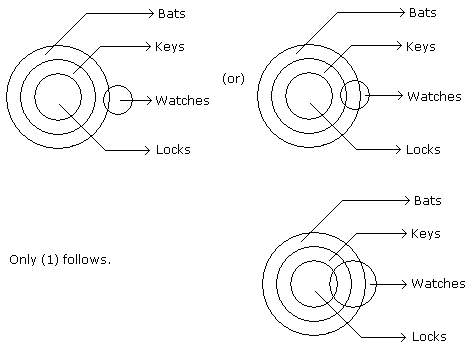QUESTION: 25

Directions to Solve

In each of the following questions there are three statements. Which are followed by three or four conclusions. Choose the conclusions which logically follow from the given statements.

Question -

Statements: Some keys are staplers. Some staplers are stickers. All the stickers are pens.

Conclusions:

1. Some pens are staplers.
2. Some stickers are keys.
3. No sticker is key.
4. Some staplers are keys.
Solution: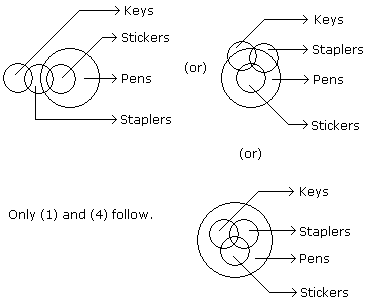QUESTION: 26

Directions to Solve

In each of the following questions there are three statements. Which are followed by three or four conclusions. Choose the conclusions which logically follow from the given statements.

Question -

Statements: Some questions are answers. Some answers are writers. All the writers are poets.

Conclusions:

2. Some poets are questions.
3. All the questions are poets.
Solution: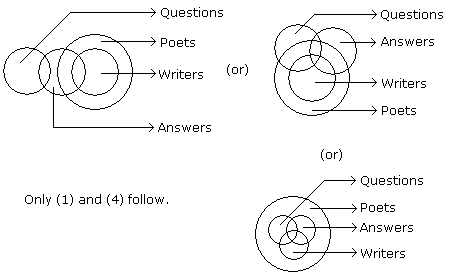QUESTION: 27

Directions to Solve

In each of the following questions there are three statements. Which are followed by three or four conclusions. Choose the conclusions which logically follow from the given statements.

Question -

Statements: Some envelops are gums. Some gums are seals. Some seals are adhesives.

Conclusions:

1. Some envelopes are seals.
Solution: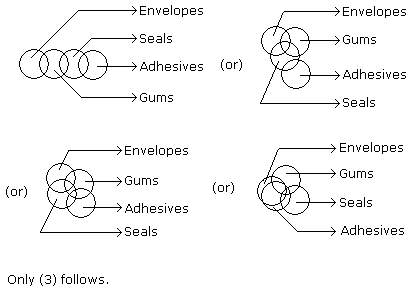QUESTION: 28

Directions to Solve

In each of the following questions there are three statements. Which are followed by three or four conclusions. Choose the conclusions which logically follow from the given statements.

Question -

Statements: All the papers are books. All the bags are books. Some purses are bags.

Conclusions:

1. Some papers are bags.
2. Some books are papers.
3. Some books are purses.
Solution: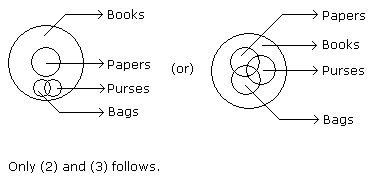QUESTION: 29

Directions to Solve

In each of the following questions there are three statements. Which are followed by three or four conclusions. Choose the conclusions which logically follow from the given statements.

Question -

Statements: Some rats are cats. Some cats are dogs. No dog is cow.

Conclusions:

1. No cow is cat.
2. No dog is rat.
3. Some cats are rats.
Solution: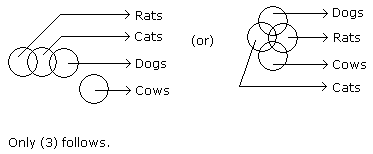QUESTION: 30

Directions to Solve

In each of the following questions there are three statements. Which are followed by three or four conclusions. Choose the conclusions which logically follow from the given statements.

Question -

Statements: All the books are papers. Some papers are journals. Some journals are calendars.

Conclusions:

1. Some journals are books.
2. Some calendars are papers.
3. Some books are journals.
4. Some books are calendars.
Solution: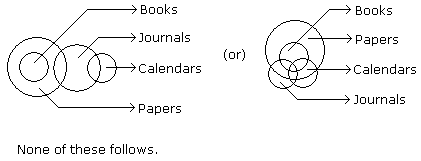Use Code STAYHOME200 and get INR 200 additional OFF Use Coupon Code